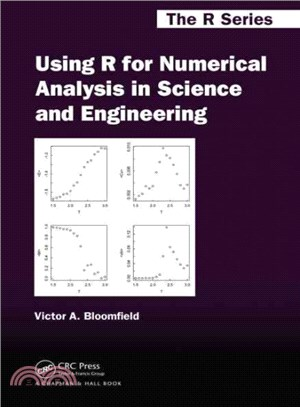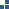瀏覽紀錄

TOP
【反詐騙】接到可疑電話該怎麼辦？提醒您「不碰不說」。聽到「訂單錯誤要操作ATM/網銀就是詐騙」！
1/1未開放訂購，若需要購買，請來電洽詢02-25006600(分機130、131)杜威分類
定  價：NT\$4725元
優惠價： 94253

未開放訂購，若需要購買，請來電洽詢02-25006600(分機130、131)商品簡介

作者簡介

Instead of presenting the standard theoretical treatments that underlie the various numerical methods used by scientists and engineers, Using R for Numerical Analysis in Science and Engineering shows how to use R and its add-on packages to obtain numerical solutions to the complex mathematical problems commonly faced by scientists and engineers. This practical guide to the capabilities of R demonstrates Monte Carlo, stochastic, deterministic, and other numerical methods through an abundance of worked examples and code, covering the solution of systems of linear algebraic equations and nonlinear equations as well as ordinary differential equations and partial differential equations. It not only shows how to use R’s powerful graphic tools to construct the types of plots most useful in scientific and engineering work, but also:

• Explains how to statistically analyze and fit data to linear and nonlinear models
• Explores numerical differentiation, integration, and optimization
• Describes how to find eigenvalues and eigenfunctions
• Discusses interpolation and curve fitting
• Considers the analysis of time series

Using R for Numerical Analysis in Science and Engineering provides a solid introduction to the most useful numerical methods for scientific and engineering data analysis using R.

Victor A. Bloomfield is currently emeritus professor at University of Minnesota, Minneapolis, USA. His research has encompassed more than four decades and a variety of topics, including enzyme kinetics, dynamic laser light scattering, bacteriophage assembly, DNA condensation, scanning tunneling microscopy, and single molecule stretching experiments on DNA. His theoretical work on biopolymer hydrodynamics and polyelectrolyte behavior has resulted in over 200 peer-reviewed journal publications. Using R for Numerical Analysis in Science and Engineering is an extension and broadening of his 2009 book, Computer Simulation and Data Analysis in Molecular Biology and Biophysics: An Introduction Using R, for general usage in science and engineering.

## 購物須知

為了保護您的權益，「三民網路書店」提供會員七日商品鑑賞期(收到商品為起始日)。

若要辦理退貨，請在商品鑑賞期內寄回，且商品必須是全新狀態與完整包裝(商品、附件、發票、隨貨贈品等)否則恕不接受退貨。

外文書商品之書封，為出版社提供之樣本。實際出貨商品，以出版社所提供之現有版本為主。部份書籍，因出版社供應狀況特殊，匯率將依實際狀況做調整。

無庫存之商品，在您完成訂單程序之後，將以空運的方式為你下單調貨。為了縮短等待的時間，建議您將外文書與其他商品分開下單，以獲得最快的取貨速度，平均調貨時間為1~2個月。